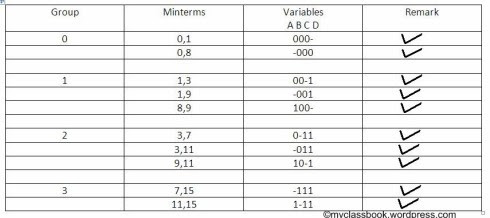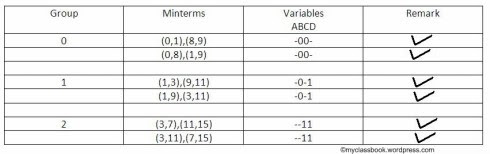# Quine Mccluskey Method with Examples

## Quine-Mcclusky method for minimization of logic function:

Question: minimize the following logic function using Quine Mcclusky method: Y(A,B,C,D) =? m(0,1,3,7,8,9,11,15)

### Table 1:

Make a table of four columns. In the first column, we have to write a group i.e. the number of ‘1’s present in given minterms. For example first group having zero times ‘1’s i.e. there is no ‘1’ in that row (see column of variables), whereas in the second group that is group having one time ‘1’, there are two possible cases first is 1=0001 and second is 8=1000 in both cases ‘1’ is present only one time. Just follow it for all the given minterms. We will discuss the remark column in the second table.

[caption id="" align="alignnone" width="490"]Quine Mccluskey Method[/caption]

Table 2:

Now here is the second table. In the second table, we have to do the same thing only the difference is that, we have to refer to the first table. For the first group i.e. group ‘0’ (0,1) and (0,8) have only one digit different (see the third column of table1). So put ‘-’ in that place. (keep in mind that group ‘0’ means there should not any ‘1’ in that row). Similarly, for group ‘1’ there should present ‘1’ only ones. Now check the remark column of the first table. When we take (0,1) for the first group, we have to fill remark column.

[caption id="" align="alignnone" width="490"]Quine Mccluskey Method[/caption]

Table 3:

The same procedure is repeated here in the third table. In this table, there will be two ‘-’s. Fill the remark column of the second table when you select minterms for next table.

[caption id="" align="alignnone" width="490"]Quine Mccluskey Method[/caption]

Table of prime implicants (PI):

Finally, the following table is of prime implicants. If you observe last table (table 3) carefully, the minterms for each group are same only the position is different, for example for first group ‘0’ there are 0,1,8,9 which is nothing but 0,8,1,9. So we have to fill prime implicants with corresponding variables of the third table. There is (-00-) it means B’C’ since A and D are absent. Similarly (-0-1) means B’D and so on.Now in the final step, we have to round those minterms which has single ‘X’ in its column. And here is our final answer i.e. corresponding PI terms which are (B’C’+CD).